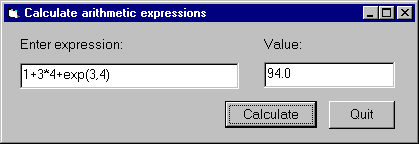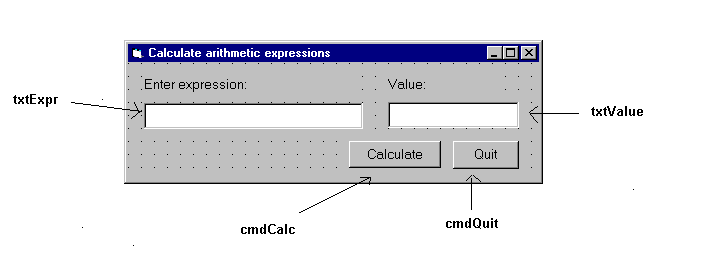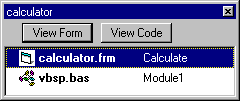46.4.1 Example 1 - Calculator

This example contains a simple program that allows you to enter an arithmetic expression (conforming to Prolog syntax) as a string and displays the value of the given expression, as shown in the following figure:The calculation itself will be done in Prolog.

We now we will go through the steps of developing this program.

1. Start a new project called `calculator`.
2. Add the vbsp.bas file to the project.
3. Create a form window called `calculator`. Edit it, adding two textboxes `txtExpr` and `txtValue`, and two command buttons, `cmdCalc` and `cmdQuit`:Save the form window to the `calculator.frm` file. Then the project will contain the following two files:4. Write the Prolog code in the file `calc.pl`, evaluating the given expression with the `is/2` predicate, and providing a minimal level of exception handling:
```          prolog_calculate(Expr, Value) :-
on_exception(Exc, Value is Expr, handler(Exc,Value)).

handler(domain_error(_,_,_,_),'Incorrect expression').
handler(Exc,Exc).
```

Note that this example focuses on a minimal implementation of the problem, more elaborate exception handling will be illustrated in the Train example (see Example 2 - Train).

Compile this file, and deposit the file calc in the directory where the `calculator.vbp` project is contained.

5. Now you have to write the Visual Basic code in which SICStus Prolog will be called at two points:
• Initialize Prolog in the `Form_Load` procedure executed when the `calc` form is loaded, calling the `PrologInit()` function and loading the calc file with the help of the `PrologQueryCutFail(..))` function:
```               Private Sub Form_Load()
If PrologInit() <> 1 Then GoTo Err
If PrologQueryCutFail("ensure_loaded(app(calc))") <> 1 Then GoTo Err
Exit Sub

Err:
End Sub
```
• Do the expression evaluation in the `calculate` procedure activated by the `cmdRun` command button. This procedure will execute the `prolog_calculate(X,Y)` procedure defined in the calc.pl Prolog file:
```               Public Function calculate(ByVal Expr As String) As String
Dim qid As Long
Dim result As String
Dim ret As Long
Dim Q As String

Q = "prolog_calculate(" & Expr & ",Value)"
qid = PrologOpenQuery(Q)
If qid = -1 Then GoTo Err ' e.g. syntax error

ret = PrologNextSolution(qid)
If ret <> 1 Then GoTo Err ' failed or error

ret = PrologGetString(qid, "Value", result)
If ret <> 1 Then GoTo Err
calculate = result
Call PrologCloseQuery(qid)

Exit Function

Err:
• Deinitialize Prolog in the `Form_Unload` procedure executed when the `calc` form is unloaded, e.g. when the application exits.
```               Private Sub Form_Unload(Cancel As Integer)